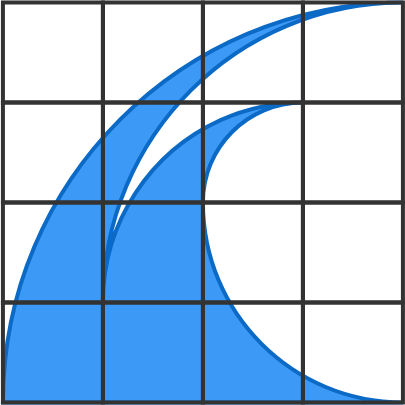An Awesome Wave

Geometry Level 2

The blue figure is made of five quarter-circle arcs (each with integer radius) and a segment of length 4, which is the base of a $4\times 4$ square grid.

What is the area of the blue figure?×